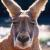python 读取数据再写入，文件大小总会出现变差？

python 2.7.8

def file_by_block(file, begin, end):
with open(file, 'rb') as fp:
fp.seek(begin)  #寻找文件中某个给定的位置
lenthofdata = end - begin  #希望读取的大小
fd.writelines(data)

def process_pid(pid, array, file, rlock):
'''
多个动作pid同时开动。
'''
FILE_SIZE = getFilesize(file)
while 1:
rlock.acquire()
begin = max(array)
end = array[pid] = (begin + BLOCKSIZE) if (begin + BLOCKSIZE) < FILE_SIZE else FILE_SIZE
rlock.release()

if begin == FILE_SIZE:
break
elif end < FILE_SIZE:
file_by_range(file, begin, end,pid)
elif end == FILE_SIZE:
file_by_range(file, begin, end,pid)
else:
break

def main():

FILE_SIZE = getFilesize(file)

rlock = RLock()
array = Array('l', WORKERS, lock=rlock)
for i in range(WORKERS):
p = Process(target=process_pid(), args=[i, array, file, rlock])

for i in range(WORKERS):

for i in range(WORKERS):

print 'in main :', datetime.datetime.now().strftime("%Y-%d-%m %H:%M:%S")

if __name__ == '__main__':
main()

0
s

1.你没有计算了不可见字符比如回车换行？

2.你的源文件不是ANSI格式，有不可见文件头。00引用来自“查询结果成为数据源”的评论